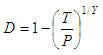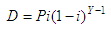# Depreciation Calculator - online spreadsheet

### Depreciation Rate and Amount

 Economic depreciation involves two different but related ideas: the decline in value of assets, and the allocation of the cost of assets to periods in which the assets are used. The first concept affects values of businesses; the second concept affects income. The depreciation calculator  below helps you study two common problems:

a) Depreciation Rate of an investment. You must provide the original price of the item, its resale price, and its age in years.
The depreciation rate is calculated by the following formula:where:

T = resale price
P = original price
Y = age
D = depreciation rate

b) Depreciation Amount. You must provide the original price, the depreciation rate and the years of depreciation. The depreciation amount is claulated by the following formula:where:

D = depreciation amount
P = original price
i = depreciation rate
Y = year of depreciation

### Calculate Depreciation Rate

Let's imagine that happy Julie bought her car for \$50,000 and sold it for only \$24,325 three years later. What was the actual depreciation rate?

We use one of the possibilities of our calculator...

Depreciation Rate
Original Price = 50,000.00
Resale Price = 24,325.00
Years = 3

Depreciation Rate =     21.35%

### Calculate Depreciation Amount

If Julie's computer had an original price of \$4,933.76. This particular high-tech laptop depreciates at an average annual rate of 21%. What amount has the laptop depreciated in the first two years?

We use now the other section of our calculator...

Depreciation Amount
Original Price =  4,933.76
Depreciation rate =  21.00
Year of depreciation = 1

Depreciation this year = 1,036.09

We just change the year of depreciation in the spreadsheet

Year of depreciation = 2

Depreciation this year = 818.51

 You have to enable JavaScript codes on this site if you want to run the calculator, otherwise it won't work.

 Enter the needed values (do not use commas) Depreciation Amount in a specific year... Original Price: Depreciation Rate: % Year of depreciation: Depreciation this year: Depreciation Rate Original Price: Resale Price: Years: Depreciation Rate: %

From 'Depreciation Calculator' to home

From 'Depreciation Calculator' to Free Online Calculators